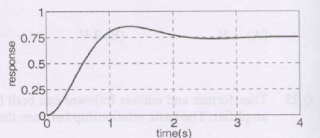## Control System Objective Questions from Competitive Exams (GATE, IES)

 The first two rows of Routh’s tabulation of a third order equation are as follows.
s3  2  2
s2  4  4
This means there are [GATE 2009]
(a) Two roots at s =± j and one root in right half s-plane
(b) Two roots at s =± j2 and one root in left half s- plane
(c) Two roots at s = ±j2 and one root in right half s-plane
(d) Two  roots at s =± j and one root in left half s-plane

 The asymptotic approximation of the log magnitude vs frequency plot of a system containing only real poles and zeros is shown. Its transfer function is [GATE 2009](a) [10(s+5)]/[s(s+2)(s+25)]

(b) [1000(s+5)]/[s2(s+2)(s+25)]
(c) [100(s+5)]/[s(s+2)(s+25)]
(d) [80(s+5)]/[s2(s+2)(s+25)]

 The trace and determinant of a 2x2 matrix are known to be -2 and -35 respectively. Its eigen values are [GATE 2009]
(a) -30 and -5
(b) -37 and -1
(c) -7 and 5
(d) 17.5 and -2
 A  Linear Time Invariant system with an impulse response h(t) produces output y(t) when input x(t) is applied. When the input x(t-τ) is applied to a system with impulse response h(t-τ), the output will be [GATE 2009]
(a) y(t)
(b) y(2(t-τ))
(c) y(t-τ)
(d) y(t-2τ)

 For the Y-bus matrix of a 4-bus system given in per unit, the buses having shunt elements are [GATE 2009](a)  3 and 4
(b)  2 and 3
(c)  1 and 2
(d)  1,2 and 4

 The unit step response of a unity feedback system with open loop transfer function G(s) = K / ((s+1)(s +2)) is shown in the figure. The value of K is
[GATE 2009](a)  0.5
(b)  2
(c)  4
(d)  6

[7For the driving point impedance function, Z(s)=[as2+7s+3]/[s2+3s+b], the circuit realization is shown below. The values of 'a' and 'b' respectively are [IES2010]

(a) 4 and 5
(b) 2 and 5
(c) 2 and 1
(d) 2 and 3

 For the following driving point impedance functions, which of the following statements is true? [IES2010]
Z1(s)=(s+2)/(s2+3s+5)
Z2(s)=(s+2)/(s2+5)
Z3(s)=(s+2)/(s2+2s+1)
Z4(s)=(s+2)(s+4)/(s+1)(s+3)
(a) Zis not positive real
(b) Z1 is positive real
(c) Z3 is positive real
(d) Zis positive real

 The steady state error of a unity feedback linear system for a unit step input is 0.1. The steady state error of the same system, for a pulse input r(t) having a magnitude of 10 and a duration of one second, as shown in the figure is [GATE2011]
(a) 0
(b) 0.1
(c) 1
(d) 10

 A point z has been plotted in the complex plane, as shown in figure below [GATE2011]

The plot of the complex number y=1/z is...........The options A,B,C,D are given below.

3:25 PM